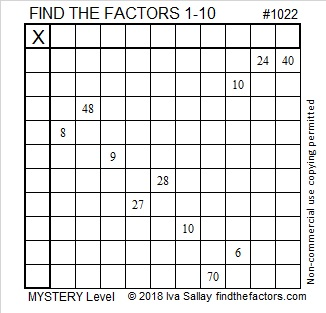# 1022 Friedman Number Mystery

1022 is the 15th Friedman number. “What is a Friedman number and why is 1022 one of them?” you may ask. I will solve that little mystery for you. 1022 is a Friedman number because
2¹⁰ – 2 = 1022. Notice that the expression 2¹⁰ – 2 uses the digits 1, 0, 2, and 2 in some order and a subtraction sign. A Friedman number can be written as an expression that uses all of its own digits the exact number of times that they occur in the number. The expression must include at least one operator (+, -, ×, ÷) or a power. Parenthesis are allowed as long as the other rules are followed.

Now I would like you to solve the mystery of this puzzle using logic and the multiplication facts. Can you do it?Print the puzzles or type the solution in this excel file: 10-factors-1019-1027

1022 is the hypotenuse of a Pythagorean triple:
672-770-1022 which is 14 times (48-55-73)

• 1022 is a composite number.
• Prime factorization: 1022 = 2 × 7 × 73
• The exponents in the prime factorization are 1, 1, and 1. Adding one to each and multiplying we get (1 + 1)(1 + 1)(1 + 1) = 2 × 2 × 2 = 8. Therefore 1022 has exactly 8 factors.
• Factors of 1022: 1, 2, 7, 14, 73, 146, 511, 1022
• Factor pairs: 1022 = 1 × 1022, 2 × 511, 7 × 146, or 14 × 73
• 1022 has no square factors that allow its square root to be simplified. √1022 ≈ 31.96873## 2 thoughts on “1022 Friedman Number Mystery”

1.Heidi Abate

I’m so happy I found this site. I’m really enjoying playing with this puzzles and think they will be great for my 7th graders. I’m wondering if you ever post the answers. I’m struggling with #1022 and can’t figure out where I’m going wrong.

•ivasallay

The secret to beginning puzzle #1022 is clue 48. Yes, it has to be either 6 x 8 or 8 x 6, but only one of them will work with the other clues. Putting 8 in the 1st column and 6 in the top row doesn’t work because then there won’t be any factors for clues (24, 40) that don’t duplicate either the 8 in the 1st column or the 6 in the top row. Thus 48 must have the 6 in the 1st column and 8 in the top row. It is pretty much straightforward to find all the other factors in the puzzle.

If you need any other hints, let me know.

This site uses Akismet to reduce spam. Learn how your comment data is processed.